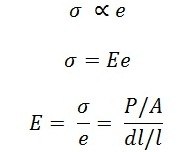# What is Hooke’s Law? – Strength of Materials

What is Hooke’s law? It is the general question that is asked in the subject strength of materials. This law gives us a relation between stress and strain. It was given by Robert Hooke in 1660. It is defined on
elastic materials.

## What is Hooke’s Law### Statement:

According to Hooke’s law, when a material is loaded within elastic limit, the stress induced in the material is directly proportional to the strain produced. It means that the ratio of stress with the corresponding strain gives us a constant within elastic limit. The constant is known as Modulus of Elasticity or Modulus of Rigidity or Elastic Modulii.

In the Stress strain curve the proportionality limit indicates the Hooke’s law. The curve shows linearity within elastic limit. The ratio of stress and corresponding strain in the stress strain curve gives us Young’s Modulus

Mathematically### Young’s Modulus or Modulus of Rigidity or Elastic Modulii.

It is defined as the ratio of stress to the strain within elastic limit. It is denoted by the letter ‘E’.

• It represents the elastic property of a material.
• The unit of Young’s modulus is N/m2.
or N/mm2. The unit of young’s modulus is same as that of units of stress.

Where,

• σ = Stress
• e = Strain
• E = Proportionality constant called as Young’s Modulus

This is all about what is Hooke’s law? If you found this piece of information useful and valuable then don’t forget to like and share it.